# MCQs on Electric Circuits

##### Page 44 of 63. Go to page 1 2 3 4 5 6 7 8 9 10 11 12 13 14 15 16 17 18 19 20 21 22 23 24 25 26 27 28 29 30 31 32 33 34 35 36 37 38 39 40 41 42 43 44 45 46 47 48 49 50 51 52 53 54 55 56 57 58 59 60 61 62 63
01․ A current in a circuit is given by,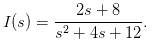If the current flows through the 5 Ω resistor, find power dissipated at t = 0?
20 Watts.
15 Watts.
40 Watts.
10 Watts.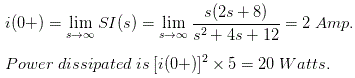02․ A circuit with resistor, inductor, capacitor in series is resonant of 50 Hz. If all the values are now doubled, the new resonant frequency is
f0 / 2.
f0 / 4.
2f0.
still f0.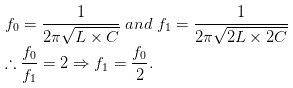03․ Can a 250 V, 5 A single way switch be used in place of a 250 V, 15 A Switch?
Single way switch doesn exist.
Yes.
No.
Single way switch doesn operate at such current ratings.

The contact strip will melt if current more than 5 A flows in 250 V, 5 A rated switch .

04․ A periodic voltage having the Fourier series V(t)= {1 + 4sinω0t}
1 W.
21 W.
11 W.
24.5 W.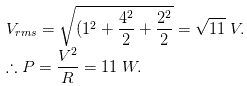05․ For the resonance circuit ω0 = 105, Q = 50, R = 400 Ω the value of C is
250 pF.
500 pF.
1000 pF.
125 pF.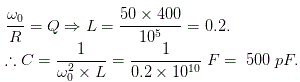06․ An AC voltage of 200 V at 50 Hz is applied to a coil which draws 5 A and dissipates 1000 W. The resistance and impedance of the coil respectively are
40 Ω and 200 Ω.
40 Ω and 40 Ω.
25 Ω and 5 Ω.
50 Ω and 200 Ω.

P = 1000W; I2R = 1000W; R = 1000/52 = 40 Ohm V = 200V; I = 5A; Z = V/I = 200/5 =40 Ohm

07․ In what connection we get neutral?
Star.
Delta.
Mesh.
Both A and B.

Star connection is a method of connecting 3 - phase circuit in such a way that one end of each phase of 3 - phase are connected together thus forming a common star point called neutral.

08․ What is the relation between line voltage and phase voltage in case of delta connection?
VL = VP.
VL = 1/ √3 VP.
VL = √3 VP.
None of these.

In case of delta connection, there is no neutral and so the phase voltage Vph = VAC, which is the line voltage as is evident from the below figure.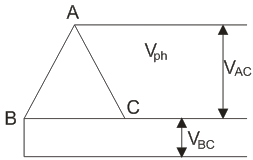09․ A capacitor of 50 microfarad is connected in series with a resistance of 120 Ω. If above circuit is connected across 240 V, 50 Hz 1 - φ supply. Find the capacitive reactance?
63.7 Ω.
0.015 Ω.
135.86 Ω.
1.765 Ω.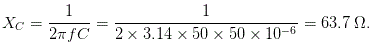10․ Link in network theory refers to
B - N + 1.
B - N - 1.
N - 1.
N - B - 1.

Link / chord is the branch of a graph that does not belong to the particular tree. It is simply called a link. Let B be branch, N be nodes. Then twig = N -1. Link, L = B -( N - 1) = B - N + 1.

<<<4243444546>>>